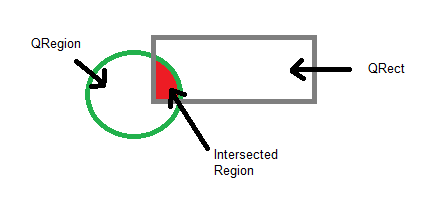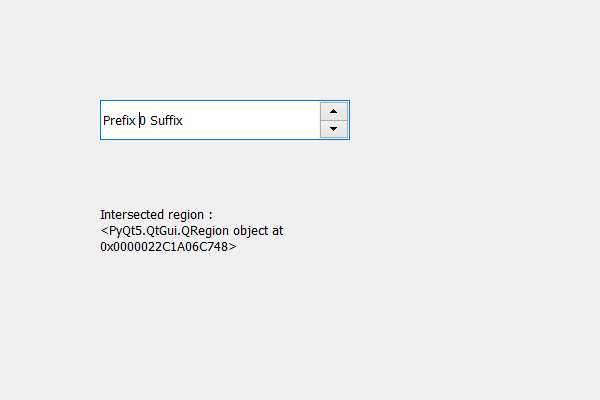# PyQt5 QSpinBox – Getting the intersected region

• Last Updated : 19 May, 2020

In this article we will see how we can get the intersected region between children region of spin box and the given rectangle or region, children region holds the combined region occupied by the spin box’s children. In order to get the children region we use `childrenRegion` method. Below is how intersected region looks like.In order to do this we use the intersected method with the children region object of the spin box

Syntax : children_region.intersected(rectangle)

Argument : It takes QRect object as argument also it can take QRegion object

Return : It returns QRegion object

Below is the implementation

 `# importing libraries``from` `PyQt5.QtWidgets ``import` `*` `from` `PyQt5 ``import` `QtCore, QtGui``from` `PyQt5.QtGui ``import` `*` `from` `PyQt5.QtCore ``import` `*` `import` `sys`` ` ` ` `class` `Window(QMainWindow):`` ` `    ``def` `__init__(``self``):``        ``super``().__init__()`` ` `        ``# setting title``        ``self``.setWindowTitle(``"Python "``)`` ` `        ``# setting geometry``        ``self``.setGeometry(``100``, ``100``, ``600``, ``400``)`` ` `        ``# calling method``        ``self``.UiComponents()`` ` `        ``# showing all the widgets``        ``self``.show()`` ` `        ``# method for widgets``    ``def` `UiComponents(``self``):``        ``# creating spin box``        ``self``.spin ``=` `QSpinBox(``self``)`` ` `        ``# setting geometry to spin box``        ``self``.spin.setGeometry(``100``, ``100``, ``250``, ``40``)`` ` `        ``# setting range to the spin box``        ``self``.spin.setRange(``0``, ``999999``)`` ` `        ``# setting prefix to spin``        ``self``.spin.setPrefix(``"Prefix "``)`` ` `        ``# setting suffix to spin``        ``self``.spin.setSuffix(``" Suffix"``)`` ` `        ``# getting the children region``        ``children_region ``=` `self``.spin.childrenRegion()`` ` `        ``# creating a label``        ``label ``=` `QLabel(``self``)``        ``label.setWordWrap(``True``)`` ` `        ``# setting geometry to the label``        ``label.setGeometry(``100``, ``200``, ``200``, ``60``)`` ` `        ``# QRect``        ``rectangle ``=` `QRect(``100``, ``100``, ``20``, ``30``)`` ` `        ``# getting intersected region between the Qrect and children region``        ``intersection_region ``=` `children_region.intersected(rectangle)`` ` `        ``# setting text to the label``        ``label.setText(``"Intersected region : "` `+` `str``(intersection_region))`` ` ` ` ` ` `# create pyqt5 app``App ``=` `QApplication(sys.argv)`` ` `# create the instance of our Window``window ``=` `Window()``window.spin.setFocus()``# start the app``sys.exit(App.``exec``())`

Output :My Personal Notes arrow_drop_up